# 我又闲下来了，再做论坛网络状态测试~~~参与者都有分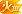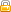[复制链接]
 香港PCCW  8M  ADSL C:\Users\Administrator>ping www.pceva.com.cn -n 20 正在 Ping pceva.3322.org [180.166.1.70] 具有 32 字节的数据: 来自 180.166.1.70 的回复: 字节=32 时间=44ms TTL=240 来自 180.166.1.70 的回复: 字节=32 时间=45ms TTL=240 来自 180.166.1.70 的回复: 字节=32 时间=46ms TTL=240 来自 180.166.1.70 的回复: 字节=32 时间=45ms TTL=240 来自 180.166.1.70 的回复: 字节=32 时间=43ms TTL=240 请求超时。 来自 180.166.1.70 的回复: 字节=32 时间=46ms TTL=240 来自 180.166.1.70 的回复: 字节=32 时间=46ms TTL=240 来自 180.166.1.70 的回复: 字节=32 时间=46ms TTL=240 来自 180.166.1.70 的回复: 字节=32 时间=45ms TTL=240 来自 180.166.1.70 的回复: 字节=32 时间=44ms TTL=240 来自 180.166.1.70 的回复: 字节=32 时间=45ms TTL=240 来自 180.166.1.70 的回复: 字节=32 时间=46ms TTL=240 来自 180.166.1.70 的回复: 字节=32 时间=44ms TTL=240 来自 180.166.1.70 的回复: 字节=32 时间=45ms TTL=240 来自 180.166.1.70 的回复: 字节=32 时间=47ms TTL=240 来自 180.166.1.70 的回复: 字节=32 时间=44ms TTL=240 来自 180.166.1.70 的回复: 字节=32 时间=47ms TTL=240 来自 180.166.1.70 的回复: 字节=32 时间=46ms TTL=240 来自 180.166.1.70 的回复: 字节=32 时间=44ms TTL=240 180.166.1.70 的 Ping 统计信息:     数据包: 已发送 = 20，已接收 = 19，丢失 = 1 (5% 丢失)， 往返行程的估计时间(以毫秒为单位):     最短 = 43ms，最长 = 47ms，平均 = 45ms 測了4次  有兩次 都掉包了 。。。神馬情況

### 评分

 wuzhipeng 发表于 2012-8-19 01:31香港PCCW  8M  ADSL C:\Users\Administrator>ping www.pceva.com.cn -n 20 PCCW的网络应该是香港最好的了吧，反正我是这么认为的。 关于丢包，你不用担心，我认为肯定是骨干网故意的，具体原因我不清楚。 我们公司在香港有办公室，PCCW的数据中心我们也租过柜子，因此，对于上海到香港的通讯，我做过长期的观察，用电信线路ping香港，延迟很低，和你的差不多，但就是有丢包，很规律性的丢包；但用联通线路PING香港，就很好，基本上不丢包。所以我基本都是用联通线路来和香港做VPN通道。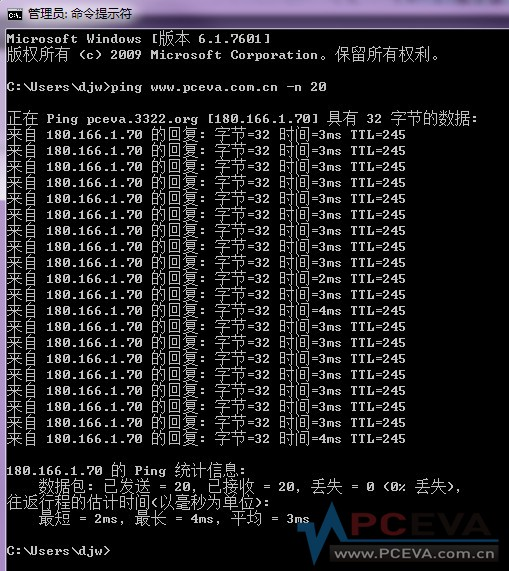上海FTTH 20M 深夜党

### 评分

 昆明联通4Ｍ。 ping bbs.pceva.com.cn -n 20 正在 Ping diablofc.3322.org [123.232.123.199] 具有 32 字节的数据: 来自 123.232.123.199 的回复: 字节=32 时间=59ms TTL=54 来自 123.232.123.199 的回复: 字节=32 时间=61ms TTL=54 来自 123.232.123.199 的回复: 字节=32 时间=59ms TTL=54 来自 123.232.123.199 的回复: 字节=32 时间=60ms TTL=54 来自 123.232.123.199 的回复: 字节=32 时间=60ms TTL=54 来自 123.232.123.199 的回复: 字节=32 时间=59ms TTL=54 来自 123.232.123.199 的回复: 字节=32 时间=60ms TTL=54 请求超时。 来自 123.232.123.199 的回复: 字节=32 时间=59ms TTL=54 来自 123.232.123.199 的回复: 字节=32 时间=60ms TTL=54 来自 123.232.123.199 的回复: 字节=32 时间=60ms TTL=54 来自 123.232.123.199 的回复: 字节=32 时间=59ms TTL=54 来自 123.232.123.199 的回复: 字节=32 时间=61ms TTL=54 来自 123.232.123.199 的回复: 字节=32 时间=60ms TTL=54 来自 123.232.123.199 的回复: 字节=32 时间=61ms TTL=54 来自 123.232.123.199 的回复: 字节=32 时间=59ms TTL=54 来自 123.232.123.199 的回复: 字节=32 时间=62ms TTL=54 来自 123.232.123.199 的回复: 字节=32 时间=61ms TTL=54 来自 123.232.123.199 的回复: 字节=32 时间=60ms TTL=54 来自 123.232.123.199 的回复: 字节=32 时间=60ms TTL=54 123.232.123.199 的 Ping 统计信息:     数据包: 已发送 = 20，已接收 = 19，丢失 = 1 (5% 丢失)， 往返行程的估计时间(以毫秒为单位):     最短 = 59ms，最长 = 62ms，平均 = 60ms

### 评分

 联通线路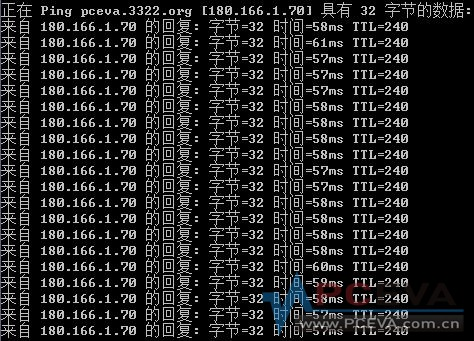### 评分

 4kfree 发表于 2012-8-18 21:37西安电信 呵呵，家人在线电影～～
 绕大半个地球稳定性还不错，比网游服务器给力。 C:\Users\       >ping www.pceva.com.cn -n 20 正在 Ping pceva.3322.org [180.166.1.70] 具有 32 字节的数据: 来自 180.166.1.70 的回复: 字节=32 时间=319ms TTL=226 来自 180.166.1.70 的回复: 字节=32 时间=319ms TTL=226 来自 180.166.1.70 的回复: 字节=32 时间=319ms TTL=226 来自 180.166.1.70 的回复: 字节=32 时间=319ms TTL=226 来自 180.166.1.70 的回复: 字节=32 时间=320ms TTL=226 来自 180.166.1.70 的回复: 字节=32 时间=319ms TTL=226 来自 180.166.1.70 的回复: 字节=32 时间=319ms TTL=226 来自 180.166.1.70 的回复: 字节=32 时间=320ms TTL=226 来自 180.166.1.70 的回复: 字节=32 时间=319ms TTL=226 来自 180.166.1.70 的回复: 字节=32 时间=321ms TTL=226 来自 180.166.1.70 的回复: 字节=32 时间=319ms TTL=226 来自 180.166.1.70 的回复: 字节=32 时间=320ms TTL=226 来自 180.166.1.70 的回复: 字节=32 时间=320ms TTL=226 来自 180.166.1.70 的回复: 字节=32 时间=319ms TTL=226 来自 180.166.1.70 的回复: 字节=32 时间=320ms TTL=226 来自 180.166.1.70 的回复: 字节=32 时间=319ms TTL=226 来自 180.166.1.70 的回复: 字节=32 时间=320ms TTL=226 来自 180.166.1.70 的回复: 字节=32 时间=320ms TTL=226 来自 180.166.1.70 的回复: 字节=32 时间=319ms TTL=226 来自 180.166.1.70 的回复: 字节=32 时间=320ms TTL=226 180.166.1.70 的 Ping 统计信息:     数据包: 已发送 = 20，已接收 = 20，丢失 = 0 (0% 丢失)， 往返行程的估计时间(以毫秒为单位):     最短 = 319ms，最长 = 321ms，平均 = 319ms

### 评分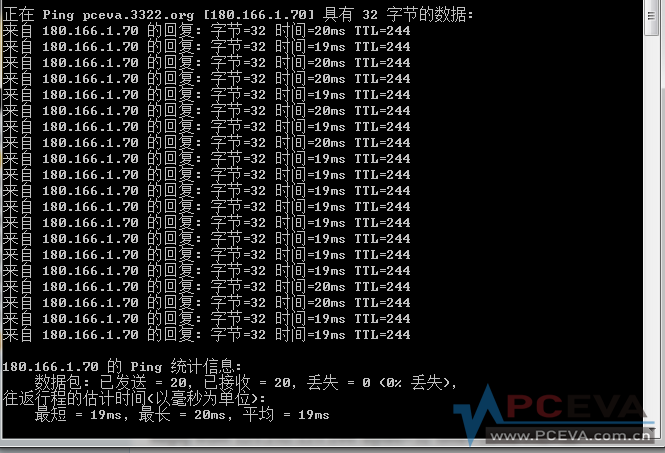福州电信20M的

### 评分

 ping服务器好玩么？
 Microsoft Windows [版本 6.1.7601] 版权所有 (c) 2009 Microsoft Corporation。保留所有权利。 C:\Users\user>ping bbs.pceva.com.cn -n 20 正在 Ping pceva.3322.org [180.166.1.70] 具有 32 字节的数据: 来自 180.166.1.70 的回复: 字节=32 时间=27ms TTL=243 来自 180.166.1.70 的回复: 字节=32 时间=27ms TTL=243 来自 180.166.1.70 的回复: 字节=32 时间=27ms TTL=243 来自 180.166.1.70 的回复: 字节=32 时间=27ms TTL=243 来自 180.166.1.70 的回复: 字节=32 时间=27ms TTL=243 来自 180.166.1.70 的回复: 字节=32 时间=27ms TTL=243 来自 180.166.1.70 的回复: 字节=32 时间=27ms TTL=243 来自 180.166.1.70 的回复: 字节=32 时间=27ms TTL=243 来自 180.166.1.70 的回复: 字节=32 时间=27ms TTL=243 来自 180.166.1.70 的回复: 字节=32 时间=27ms TTL=243 来自 180.166.1.70 的回复: 字节=32 时间=27ms TTL=243 来自 180.166.1.70 的回复: 字节=32 时间=27ms TTL=243 来自 180.166.1.70 的回复: 字节=32 时间=27ms TTL=243 来自 180.166.1.70 的回复: 字节=32 时间=27ms TTL=243 来自 180.166.1.70 的回复: 字节=32 时间=27ms TTL=243 来自 180.166.1.70 的回复: 字节=32 时间=28ms TTL=243 来自 180.166.1.70 的回复: 字节=32 时间=27ms TTL=243 来自 180.166.1.70 的回复: 字节=32 时间=27ms TTL=243 来自 180.166.1.70 的回复: 字节=32 时间=27ms TTL=243 来自 180.166.1.70 的回复: 字节=32 时间=27ms TTL=243 180.166.1.70 的 Ping 统计信息:     数据包: 已发送 = 20，已接收 = 20，丢失 = 0 (0% 丢失)， 往返行程的估计时间(以毫秒为单位):     最短 = 27ms，最长 = 28ms，平均 = 27ms C:\Users\user>

### 评分

 Microsoft Windows [版本 6.1.7601] 版权所有 (c) 2009 Microsoft Corporation。保留所有权利。 C:\Users\Jerome>ping 180.166.1.70 -n 20 正在 Ping 180.166.1.70 具有 32 字节的数据: 来自 180.166.1.70 的回复: 字节=32 时间=32ms TTL=241 来自 180.166.1.70 的回复: 字节=32 时间=31ms TTL=241 来自 180.166.1.70 的回复: 字节=32 时间=31ms TTL=241 来自 180.166.1.70 的回复: 字节=32 时间=31ms TTL=241 来自 180.166.1.70 的回复: 字节=32 时间=32ms TTL=241 来自 180.166.1.70 的回复: 字节=32 时间=32ms TTL=241 来自 180.166.1.70 的回复: 字节=32 时间=31ms TTL=241 来自 180.166.1.70 的回复: 字节=32 时间=31ms TTL=241 来自 180.166.1.70 的回复: 字节=32 时间=31ms TTL=241 来自 180.166.1.70 的回复: 字节=32 时间=31ms TTL=241 来自 180.166.1.70 的回复: 字节=32 时间=31ms TTL=241 来自 180.166.1.70 的回复: 字节=32 时间=31ms TTL=241 来自 180.166.1.70 的回复: 字节=32 时间=31ms TTL=241 来自 180.166.1.70 的回复: 字节=32 时间=32ms TTL=241 来自 180.166.1.70 的回复: 字节=32 时间=32ms TTL=241 来自 180.166.1.70 的回复: 字节=32 时间=31ms TTL=241 来自 180.166.1.70 的回复: 字节=32 时间=32ms TTL=241 来自 180.166.1.70 的回复: 字节=32 时间=31ms TTL=241 来自 180.166.1.70 的回复: 字节=32 时间=32ms TTL=241 来自 180.166.1.70 的回复: 字节=32 时间=31ms TTL=241 180.166.1.70 的 Ping 统计信息:     数据包: 已发送 = 20，已接收 = 20，丢失 = 0 (0% 丢失)， 往返行程的估计时间(以毫秒为单位):     最短 = 31ms，最长 = 32ms，平均 = 31ms C:\Users\Jerome>

### 评分

 还是联通服给力 正在 Ping diablofc.3322.org [123.232.123.199] 具有 32 字节的数据: 来自 123.232.123.199 的回复: 字节=32 时间=8ms TTL=54 来自 123.232.123.199 的回复: 字节=32 时间=9ms TTL=54 来自 123.232.123.199 的回复: 字节=32 时间=8ms TTL=54 来自 123.232.123.199 的回复: 字节=32 时间=9ms TTL=54 来自 123.232.123.199 的回复: 字节=32 时间=8ms TTL=54 来自 123.232.123.199 的回复: 字节=32 时间=9ms TTL=54 来自 123.232.123.199 的回复: 字节=32 时间=9ms TTL=54 来自 123.232.123.199 的回复: 字节=32 时间=8ms TTL=54 来自 123.232.123.199 的回复: 字节=32 时间=8ms TTL=54 来自 123.232.123.199 的回复: 字节=32 时间=8ms TTL=54 来自 123.232.123.199 的回复: 字节=32 时间=8ms TTL=54 来自 123.232.123.199 的回复: 字节=32 时间=8ms TTL=54 来自 123.232.123.199 的回复: 字节=32 时间=8ms TTL=54 来自 123.232.123.199 的回复: 字节=32 时间=8ms TTL=54 来自 123.232.123.199 的回复: 字节=32 时间=8ms TTL=54 来自 123.232.123.199 的回复: 字节=32 时间=8ms TTL=54 来自 123.232.123.199 的回复: 字节=32 时间=8ms TTL=54 来自 123.232.123.199 的回复: 字节=32 时间=9ms TTL=54 来自 123.232.123.199 的回复: 字节=32 时间=8ms TTL=54 来自 123.232.123.199 的回复: 字节=32 时间=9ms TTL=54 123.232.123.199 的 Ping 统计信息:     数据包: 已发送 = 20，已接收 = 20，丢失 = 0 (0% 丢失)， 往返行程的估计时间(以毫秒为单位):     最短 = 8ms，最长 = 9ms，平均 = 8ms

### 评分

 dy4932 发表于 2012-8-19 18:46ping服务器好玩么？ 我觉着挺好玩~~~内行看门道，外行看热闹。

### 评分

 到上海的SDH延迟也是48-50ms的样子 感觉SDH真心没意思，太贵了
 xxy 发表于 2012-8-21 09:54到上海的SDH延迟也是48-50ms的样子 感觉SDH真心没意思，太贵了 不错啦，到底跨越了大半个中国了。
 gmx168 发表于 2012-8-21 10:22不错啦，到底跨越了大半个中国了。 以后光纤材料、网络设备升级了，也只能提高几ms，光速和距离在那儿摆着，没办法的事。 不过SDH 2000块1M，商务光纤 300块1M，确实差太多。
 天津联通，2M光纤，免费升过级，现在应该是4M或是6M

ping### 评分

 本帖最后由 彩虹糖 于 2012-8-21 15:58 编辑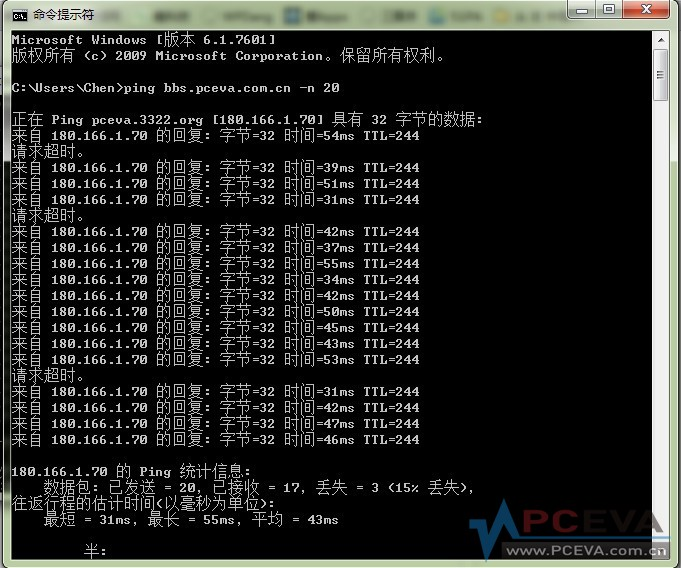公司
 您需要登录后才可以回帖 登录 | 注册 本版积分规则 回帖并转播 回帖后跳转到最后一页